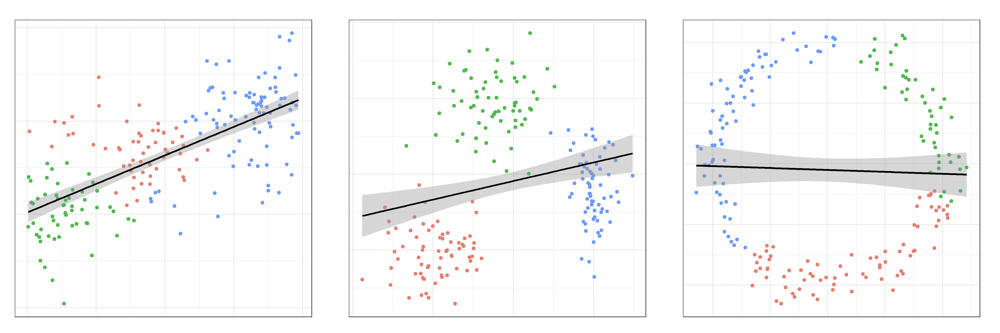## Varselmelding

There has not been added a translated version of this content. You can either try searching or go to the "area" home page to see if you can find the information there
dCod blog post

# Introducing - Topological data analysis

Understanding the structure and relationships of biomolecules is important for discovering new medicines and materials. Three-dimensional bimolecular structures are often geometrically complex making it difficult to predict functional properties of molecules based on their structures. Recently, the new field of topological data analysis has shown some promise in improving the prediction of solvation free energies, protein-ligand binding affinities and other properties.Three different datasets, each with a linear regression and a cluster analysis. Topological data analysis aims to determine what kind of further analysis may be appropriate.
Foto/ill.:
Nello Blaser

Topology is the field of mathematics that identifies essential structures of a space and quantifies qualitative shape information by stripping away the irrelevant geometrical details. An important tool in algebraic topology are the betti numbers, which encode the connectivity of a space. The first betti number counts the number of connected components, the second betti number distinct one-dimensional cycles, the third betti number distinct two-dimensional voids and further betti numbers higher-dimensional voids of a space.

A first step of data analysis is often to visualize two-dimensional summaries of the data in order to get to know the data before deciding on an analysis strategy. This works great when the data or its most important feature is indeed two-dimensional but it poses the risk of missing higher-dimensional structures in the data. It is therefore desirable to be able to summarize the most important geometric features of the data in a different way. That is where topological data analysis comes in. With persistent homology, the most important method in topological data analysis, it is possible to calculate betti numbers at different scales simultaneously and visualize them in a persistence diagram.

As an example, let us look at three different two-dimensional datasets. The first dataset was sampled from a line, the second from three clusters and the third from a circle. Of course it is possible to do cluster analysis or linear regression for all the examples. But clearly not all analyses are equally appropriate for all datasets. In the case shown below this is obvious, but when data is high-dimensional, it may not always be clear which method actually is appropriate, if any. That is when topological data analysis can provide a first impression of the data and guide the analysis to use reasonable methods.

The rise of topological data analysis has provided new insights in algebraic topology. Many recent studies have also applied persistent homology. However, for many of these studies, topological methods were not instrumental in reaching the conclusions. It remains to be seen how significant an impact topological methods have on machine learning applications.